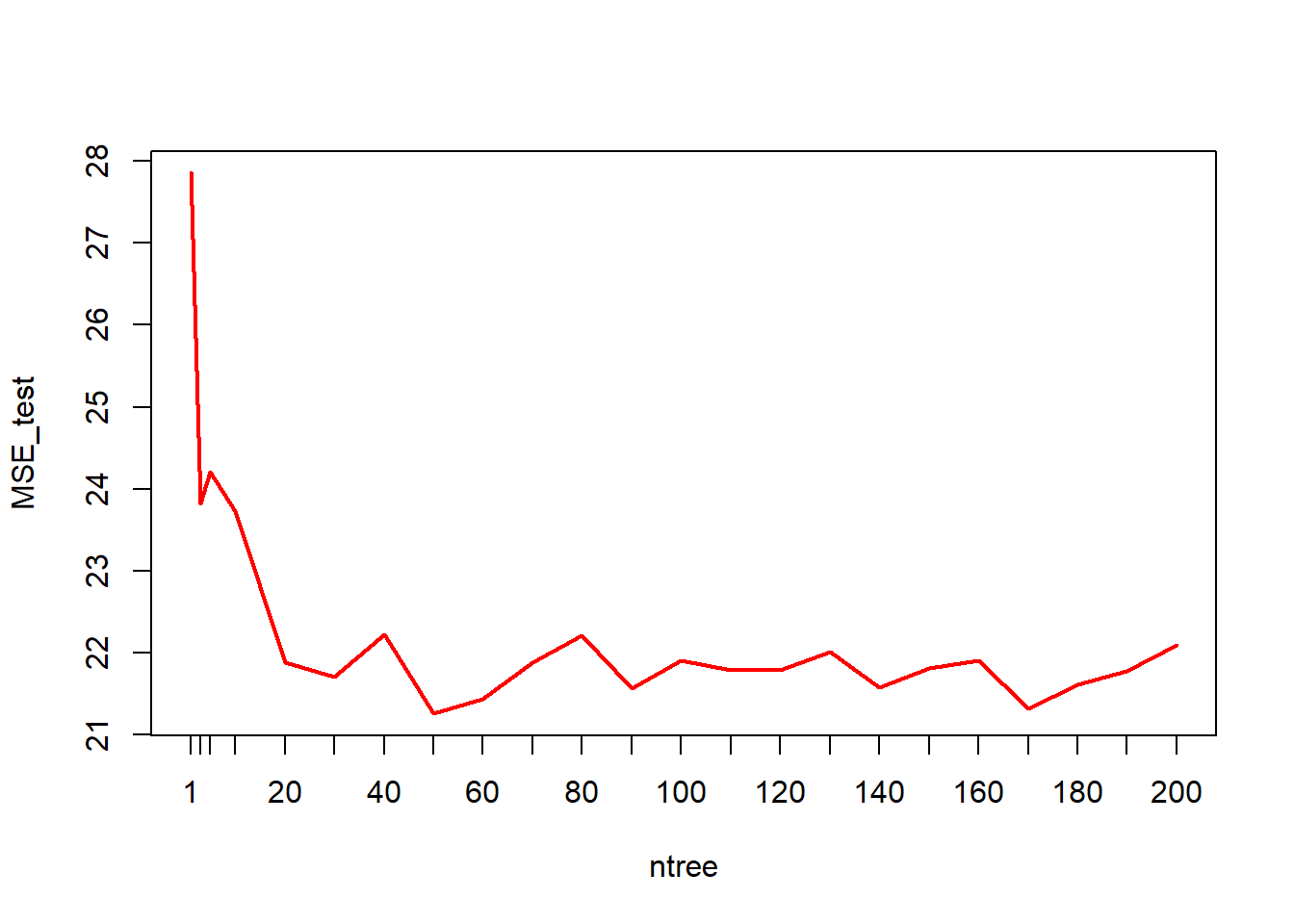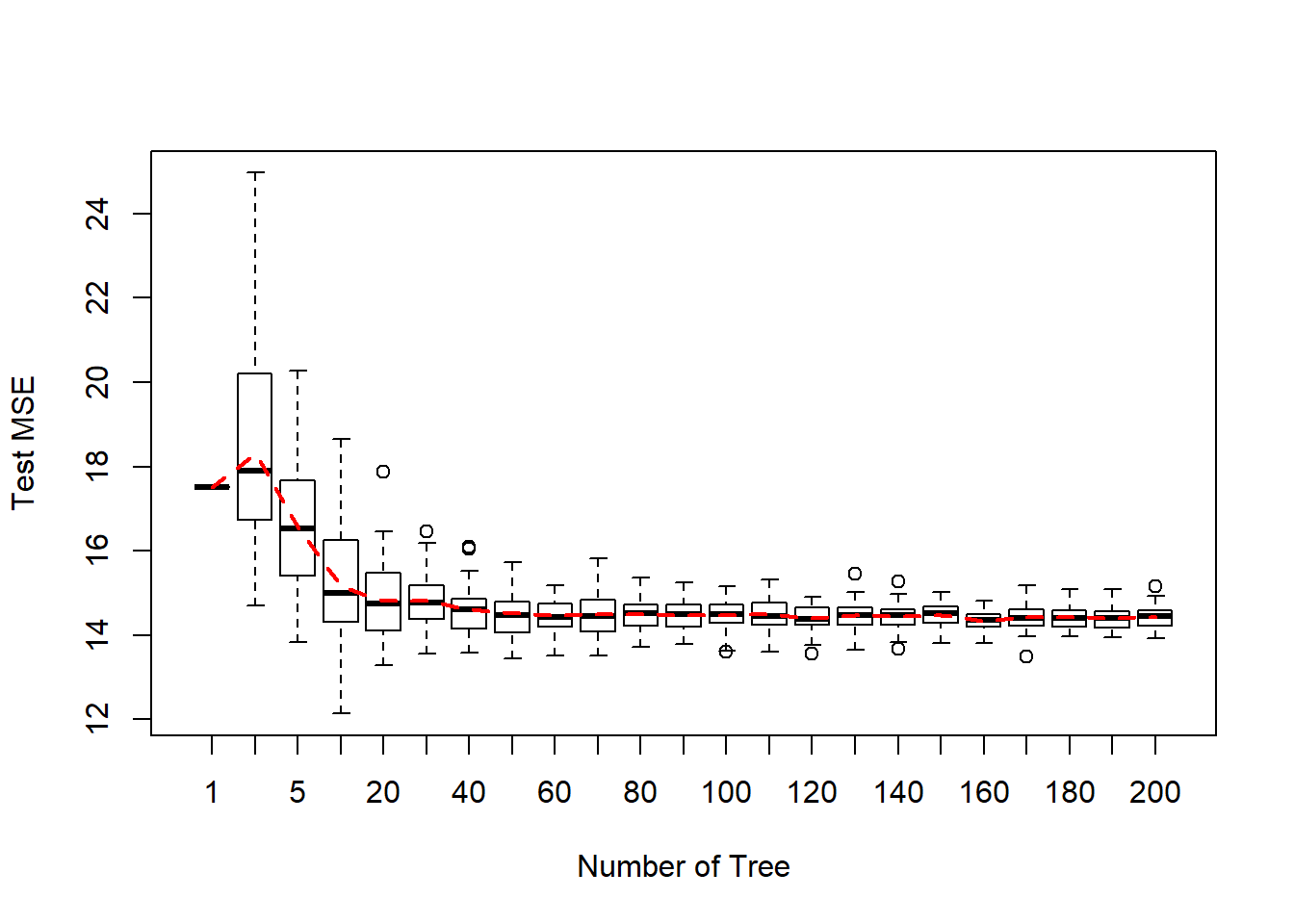In this lab, we will cover some state-of-the-art techniques in the framework of tree models. We use the same datasets as in previous lab, Boston Housing data and Credit Scoring data.

# load Boston data
library(MASS)
data(Boston)
index <- sample(nrow(Boston),nrow(Boston)*0.60)
boston_train <- Boston[index,]
boston_test <- Boston[-index,]

# convert categorical variables
credit_data$SEX<- as.factor(credit_data$SEX)
credit_data$EDUCATION<- as.factor(credit_data$EDUCATION)
credit_data$MARRIAGE<- as.factor(credit_data$MARRIAGE)
# random splitting
index <- sample(nrow(credit_data),nrow(credit_data)*0.60)
credit_train = credit_data[index,]
credit_test = credit_data[-index,]

# 1 Bagging

Bagging stands for Bootstrap and Aggregating. It employs the idea of bootstrap but the purpose is not to study bias and standard errors of estimates. Instead, the goal of Bagging is to improve prediction accuracy. It fits a tree for each bootsrap sample, and then aggregate the predicted values from all these different trees. For more details, you may look at Wikepedia, or you can find the original paper Leo Breiman (1996).

An available R package, ipred, provides functions to perform Bagging. You need to install this package if you didn’t do it before.

library(ipred)

## 1.1 Bagging for regression tree.

Fit tree with bagging on Boston training data, and calculate MSE on testing sample.

boston_bag<- bagging(formula = medv~.,
data = boston_train,
nbagg=100)
boston_bag
##
## Bagging regression trees with 100 bootstrap replications
##
## Call: bagging.data.frame(formula = medv ~ ., data = boston_train, nbagg = 100)

Prediction on testing sample.

boston_bag_pred<- predict(boston_bag, newdata = boston_test)
mean((boston_test$medv-boston_bag_pred)^2) ##  21.90055 Comparing with a single tree. library(rpart) boston_tree<- rpart(formula = medv~., data = boston_train) boston_tree_pred<- predict(boston_tree, newdata = boston_test) mean((boston_test$medv-boston_tree_pred)^2)
##  27.85463

How many trees are good?

ntree<- c(1, 3, 5, seq(10, 200, 10))
MSE_test<- rep(0, length(ntree))
for(i in 1:length(ntree)){
boston_bag1<- bagging(medv~., data = boston_train, nbagg=ntree[i])
boston_bag_pred1<- predict(boston_bag1, newdata = boston_test)
MSE_test[i]<- mean((boston_test\$medv-boston_bag_pred1)^2)
}
plot(ntree, MSE_test, type = 'l', col=2, lwd=2, xaxt="n")
axis(1, at = ntree, las=1)By fitting the Bagging multiple times and predicting the testing sample, we can draw the following boxplot to show the variance of the prediction error at different number of trees.## 1.2 Out-of-bag (OOB) prediction

The out-of-bag prediction is similar to LOOCV. We use full sample. In every bootstrap, the unused sample serves as testing sample, and testing error is calculated. In the end, OOB error, root mean squared error by default, is obtained

boston_bag_oob<- bagging(formula = medv~.,
data = boston_train,
coob=T,
nbagg=100)
boston_bag_oob
##
## Bagging regression trees with 100 bootstrap replications
##
## Call: bagging.data.frame(formula = medv ~ ., data = boston_train, coob = T,
##     nbagg = 100)
##
## Out-of-bag estimate of root mean squared error:  3.9623